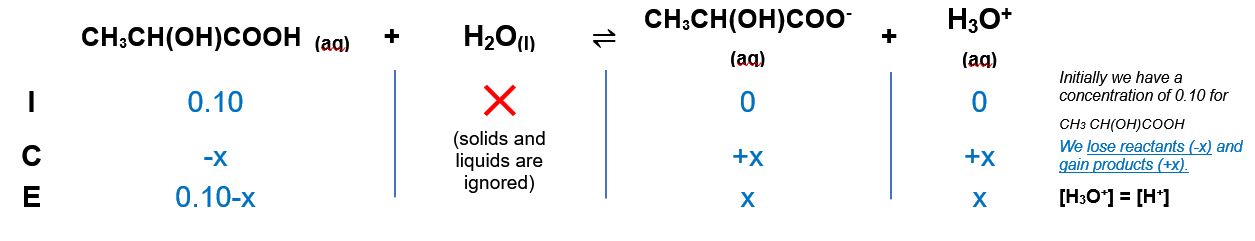# Problem: Lactic acid (CH3 CH(OH)COOH) has one acidic hydrogen. A 0.10 M solution of lactic acid has a pH of 2.44.Calculate Ka.

###### FREE Expert Solution

We’re being asked to calculate the Ka of Lactic acid (CH3CH(OH)COOH) (The pH of 0.10 M lactic acid is 2.44)

Lactic acid (CH3CH(OH)COOH) is a weak acid. Remember that weak acids partially dissociate in water and that acids donate H+ to the base (water in this case). The dissociation of Lactic Acid is as follows:

CH3CH(OH)COOH (aq) + H2O(l) CH3CH(OH)COOH(aq) + H3O+(aq)

From this, we can construct an ICE table. Remember that liquids are ignored in the ICE table.The Ka expression for CH3CH(OH)COOH  is:

Liquids are ignored in the Ka expression.

Note that each concentration is raised by the stoichiometric coefficient: [CH3CH(OH)COOH], [H3O+] and [CH3CH(OH)COO-] are raised to 1.

100% (33 ratings)###### Problem Details

Lactic acid (CH3 CH(OH)COOH) has one acidic hydrogen. A 0.10 M solution of lactic acid has a pH of 2.44.

Calculate Ka.